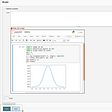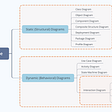# 0/1 Knapsack Problem(Memoized) — Day 42(Python)

In the previous post, we learned a few things about dynamic programming, we learned how to solve the 0/1 knapsack problem using recursion. Let us learn how to memoize the recursive solution and solve it in an optimized way.

What does memoization mean?

Let us try to find the Fibonacci series using recursion first.

`class FiboFinder:    def fibo(n):        if(n == 1):            return 1        elif(n == 0):            return 0        else:            return fibo(n-1) + fibo(n-2)`

The below image shows us the recursive calls that are made when we have to find the 5th Fibonacci term.

We can see that lot of calculations are repetitive. If we can store these calculations in some form of data structure, we can retrieve the results when needed. This form of storing the results so as retrieve the values when needed is known as memoization.

How do we decide on what needs to be memorized?

Anything that changes in values is supposed to be memoized. In our case, when we perform recursion, the value of n keeps changing. Hence we store the value of n and its result in a dictionary.

Let us see how we can perform memoization for the above code.

`class FiboFinder:    def __init__(self):        self.fibDict = dict()        self.fibDict = 0        self.fibDict = 1            def fib(self, N: int) -> int:        if(N in self.fibDict.keys()):            return self.fibDict[N]        else:            self.fibDict[N] = self.fib(N-1) + self.fib(N-2)            return self.fibDict[N]`

In the below diagram we can see that a lot of recalculations are avoided by saving in a dictionary.

Okay, let's move to the knapsack problem.

Let us recall our code for recursive solution for the knapsack problem.

`def knapSack(W, wt, val, n):    '''    :param W: capacity of knapsack     :param wt: list containing weights    :param val: list containing corresponding values    :param n: size of lists    :return: Integer    '''    # code here    if n == 0 or W == 0:        return 0    if wt[n-1] <= W:        return (max(val[n-1]+knapSack(W-wt[n-1], wt, val, n-1), knapSack(W, wt, val, n-1)))    else:        return (knapSack(W, wt, val, n-1))`

If we observe the code we understand there are 2 variables that keep changing, “W” i.e total weight of the knapsack and “n” i.e. the size of the list of items that we have. Hence we should use a data structure that can hold these two variables as well as the result when we have these 2 variables. 2-D array is a data structure that can store all these values.

Let us see how we can use a 2-D array and store the intermediate results.

In the constraints, it is provided to us that the “n”, as well as “W”, will be within the range of (1,1000). Hence a 2-D array of size 1001 is declared and initialized with -1.

Next, we need to check if the given combination of n and W is not -1. If it is not -1, it means that we have already computed the result for the given combination and hence we can directly retrieve that value. Else we save the result that we get through the recursive call to the current combination of n and W in our 2-D array and return it.

`class KnapsackFinder:    def __init__(self):        self.memo = [[-1 for i in range(1001)] for j in range(1001)]        def knapSack(W, wt, val, n):        '''        :param W: capacity of knapsack         :param wt: list containing weights        :param val: list containing corresponding values        :param n: size of lists        :return: Integer        '''        # code here        if n == 0 or W == 0:            return 0        if self.memo[n][W] != -1:            return self.memo[n][W]        if wt[n-1] <= W:            self.memo[n][W] = max(val[n-1]+knapSack(W-wt[n-1], wt, val, n-1),knapSack(W, wt, val, n-1))            return self.memo[n][W]        elif wt[n-1] > W:            self.memo[n][W] = knapSack(W, wt, val, n-1)            return self.memo[n][W]`

Complexity analysis.

Time Complexity

Since we are avoiding duplicate calculation by storing intermediate results in a 2-D array. The time complexity, in this case, is O(N*W) where N is the number of items and W is the total weight the knapsack can hold.

Space Complexity.

We are creating an array of size 1001 * 1001 and hence the space complexity is O(1001*1001).

I will be writing the third part of this problem which includes the dynamic programming approach to solve this problem.

I would love to hear your feedback about my posts. Do let me know if you have any comments or feedback.

--

--

--

## More from The Startup

Get smarter at building your thing. Follow to join The Startup’s +8 million monthly readers & +756K followers.

## eBay Event Notification Platform: Listener SDKs## Investment Portfolio Diversification By Using Data Analysis in Python## Puttering with Poetry in Mathematica## Learn TDD in Ruby in 5 easy steps## Embed Jupyter notebook into static webpages locally## The Importance of Scaling Infrastructure the Right Way## Welcome to Pluto, the Starting Place for Open Source Development## Annamariya Tharayil

Software Engineer. Find me @ www.linkedin.com/in/annamariya-jt

## CodeSignal — My favorite code challenge website.## Guide To Dynamic Programming 👨‍💻## What Is Object-Oriented Programming?## Information System: Intro. & overview